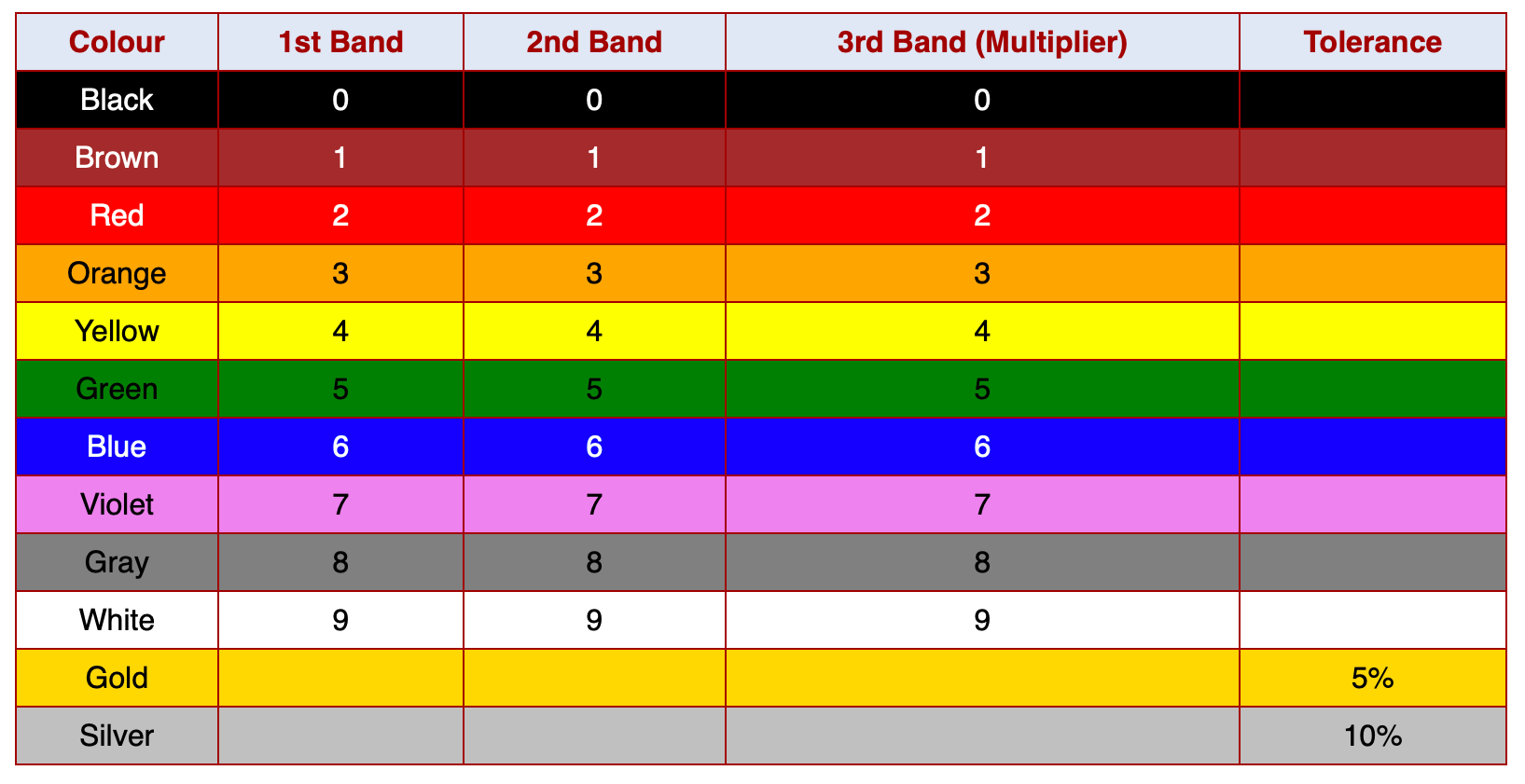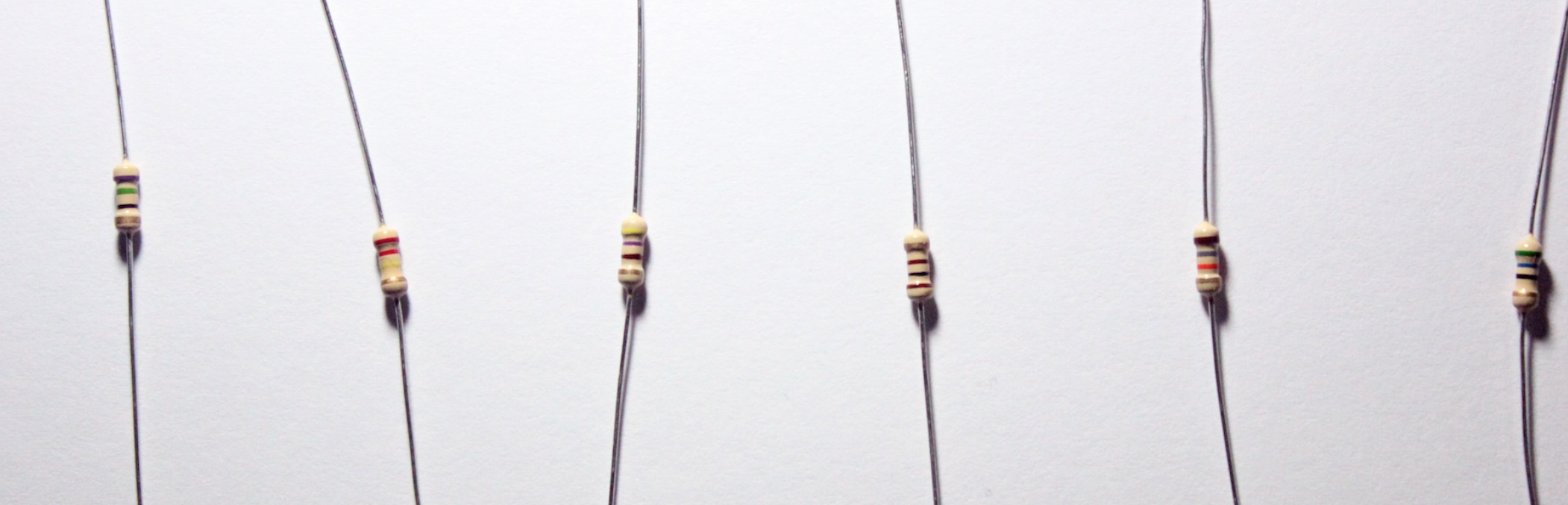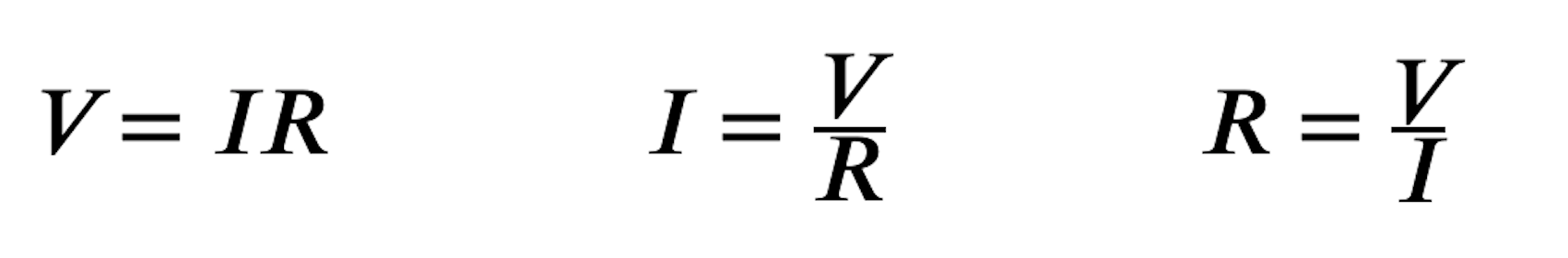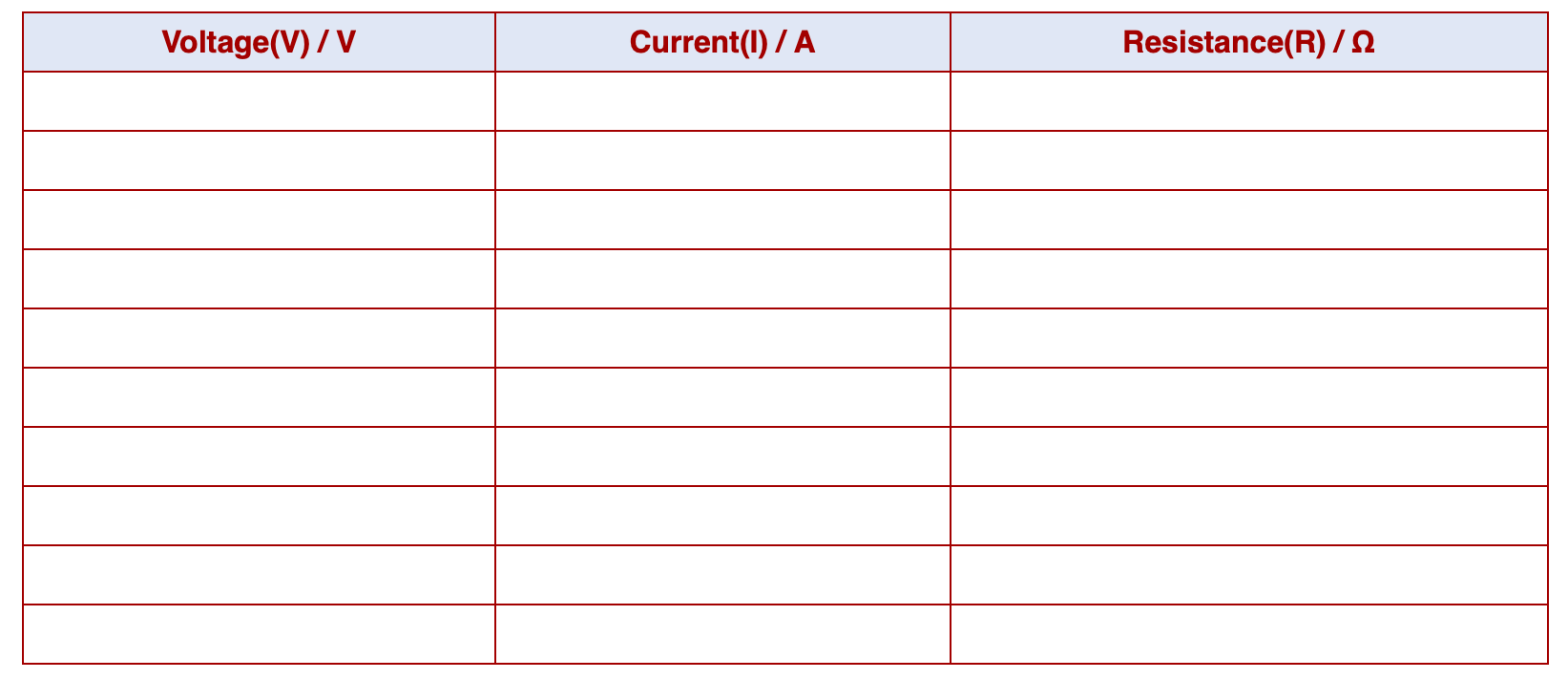# The LED Torch - Resistors and Ohm's Law

## 1 Colour Codes

### Learn It

• Resistance is measured in Ω
• You know that the narrower the wire through which electricity passes, the more the resistance it has.
• The most common type of resistors called Carbon Film resistors are made up of a carbon tube into which a grooves have been cut.
• The grooves mean there is less carbon for the current to pass through, so the resistance can be increased by having a deeper groove.### Learn It

• Resistors are colour coded so we can tell what there resistance is.
• Most resistors have 4 bands painted on the outside.• Starting at the opposite end to the Gold or Silver band (In this image that would be the right hand side):
• The first band gives us the first number
• The second band give us the second number
• The third band gives us the number of zeros.
• The fourth bands gives us the tolerance.• So for example, the resistor pictured above has the colours:
1. Brown - 1 (First number is 1)
2. Black - 0 (Second number is 0)
3. Yellow -4 (4 zeros)
4. Gold - +/-5%
• This means it is a 100000Ω resistor, or what we would normally call a 100kΩ resistor.
• The 5% tolerance means that the actual resistance can vary by + or = 5%, which in this case would be 0.05 X 100000 = 5000Ω

## 2 Assessment

#### Progress ladder Red, Identify several electronic components, with assistance.

• Look at the resistors in this photograph.• For each resistor, use the colour table to identify it's resistance.

## 3 Ohm's Law

• You've seen how increasing the resistance in a wire can effect the current passing through the wire.
• It is therefore possible to reduce the amount of current flowing in a circuit, by adding in resistors.
• To do this we use `Ohm's Law`
V=IR
• `V` is for Voltage, `I` for current and `R` is for resistance.
• We can rearange the equation to make `I` the subject, which will allow us to calculate the current in a circuit.
• Look at the animation below to see how.

• So there are three different ways of writing Ohm's Law## 4 Assessment

#### Progress ladder Orange, Identify several electronic components, without assistance.

• Copy you own version of this table shown below
• Look at the simple circuit detailed below, click on the 'get values' button for 2 of the 3 values needed to calculate the third.• Hit the button to generate a new question. Fill in the provided values into your copy of the table and then caluclate the third value for yourself.
• Sometimes the current is described in `mA`. This is short for milliamps, which are a thousandth of an amp. (Just divide it by 1000 to calculate the current in amps)